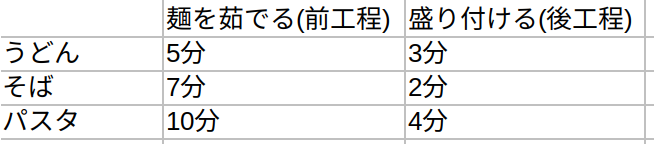# [GO] Johnson method (python)

## Johnson method

A method of finding a schedule that optimizes the work period when each product is completed through two processes.In the example of the image above, find the optimum order for boiling the noodles. Reference

## Try to write

#### `test.py`

``````

# -*- coding: utf-8 -*-
#Johnson method

def johnsonMethod(job_pair):

front = []
back = []
process_1 = []
process_2 = []
sum_time = 0

while True:

min_jobs = [min(jobs) for jobs in job_pair]
min_job = min(min_jobs)

if min_job == 9999:
sum_time += process_1 #Add the very first pre-process and finish
break

job_pair_index = min_jobs.index(min_job)
job_index = job_pair[job_pair_index].index(min_job)

if job_index == 0:
front.append(job_pair_index)
else:
back.append(job_pair_index)

process_1.append(job_pair[job_pair_index])
process_2.append(job_pair[job_pair_index])

if sum(process_1) > sum(process_2):
sum_time += job_pair[job_pair_index]
else:
sum_time += job_pair[job_pair_index]

job_pair[job_pair_index] = [9999, 9999]

front.extend(list(reversed(back)))
order = ["J" + str(x + 1) for x in front]
print(order)
print("Time required: " + str(sum_time))

#"https://studying.jp/shindanshi/past-exam/exam20unei.html 18th question"
johnsonMethod([[5,5],[6,4],[4,3],[2,8],[5,7]])
``````